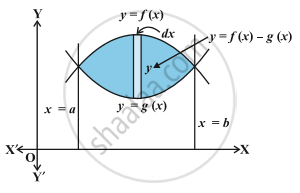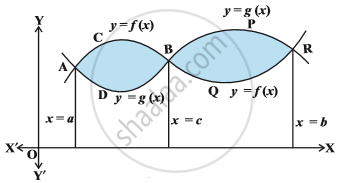# Area Between Two Curves

#### notes

The two curve represented by y = f (x), y = g (x), where f(x) ≥ g(x) in [a, b] as shown in  following Fig. Here the points of intersection of these two curves are given by x = a and x = b obtained by taking common values of y from the given equation of two curves. To take elementary area in the form of vertical strips has height f(x) - g(x) and width dx so that the elementary areadA = [f(x) – g(x)] dx, and the total area A can be taken as
A = int _a^b [f(x) - g(x)] dx , and the total area A can be taken as

Alternatively,
A = [area bounded by y = f (x), x-axis and the lines x = a, x = b] –
[area bounded by y = g (x), x-axis and the lines x = a, x = b]
= int _a^b f(x) dx -  int _a^b g(x) dx = int _a^b[f(x) - g(x)] dx , where f(x) >= g(x) in [a,b]
If f (x) ≥ g (x) in [a, c] and f (x) ≤ g (x) in [c, b], where a < c < b as shown in the following  Fig. then the area of the regions bounded by curves can be written asTotal Area = Area of the region ACBDA + Area of the region BPRQB
=int _a^c [f(x) - g(x)] dx + int _c^b [g(x) - f(x)] dx

If you would like to contribute notes or other learning material, please submit them using the button below.

### Shaalaa.com

Application of Integrals part 8 (Area between two curves) [00:14:38]
S